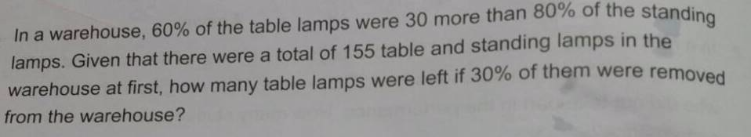# QuestionNeed Help with this question. Thanks in advance.

Just putting the solution in branch diagram.

Step 1:  Branch out a diagram and write down information:

Total lamps 155

Table  100% (     )                            Standing 100% (       )

40% =2/5 (      )              60%=3/5 (     )            80%= 4/5 (      )              20%=1/5 (    )

KIV (Keep In View):  60% of table lamps is 30 more than 80% of standing lamps

Step 2:  Compare what we need to compare

Total lamps 155

Table Lamps   100% (     )                        Standing Lamps 100% (       )

40% =2/5 (      )           60%=3/5 (     )            80%= 4/5 (      )              20%=1/5 (    )

Step 3:  “Quick and dirty” way is LCM of 3units and 4parts is 12u. “convenient” no. of u

See sequential plucking in of units.

Total lamps 155  (#7. 35u+50)

Table Lamps   100% (#6. 20u+50)                     Standing Lamps 100% (#4. 15u)

40% =2/5 (#5. 8u+20)   60%=3/5 (#2. 12u+30)     80%= 4/5 (#1. 12u)              20%=1/5 (#3. 3u)

[note for #5: working quick with %. 60% = 12u+30. Divide by 3 is 20% = 4u+10. Multiply by 2 is 40%=8u+20]

Step 4: Solve u and find answers

35u+50 = 155 –> u=3

Write down total.

Total lamps 155  (35u+50)

Table   100% (20u+50)=110                          Standing 100% (15u)=45

40% =2/5 (8u+20)     60%=3/5 (12u+30)              80%= 4/5 (12u)          20%=1/5 (3u)

44                                  66                                       36                                   9

Solve: 40% of table lamps is 44 –> 10% is 11

Remainder after 30% removed is 70% left = 77 table lamps left#

0 Replies 0 Likes

If there are 10u of table lamp
80% of standing lamp = 6u -30
Total standling lamp = (6u-30)/0.8 = 7.5u – 37.5

10u + 7.5u -37.5 = 155
17.5u = 192.5
u = 11

Number of table lamp = 10u = 110

If 30% of the table lamp were removed, there will be 70% left
0.7 X 110 = 77 left ###

0 Replies 2 Likes